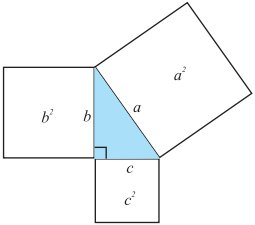# Anti-Pythagoras

Geometry Level 3In a picture such as a triangle above, we know that the areas $c^2 + b^2 = a^2$.

However, imagine a triangle with non-zero area and sides $a,b,c$ where $c$ is the longest, with squares extended outwards from each side, each with a side length of that triangle. In what cases is the sum of the perimeters of the squares $a$ and $b$ greater than the perimeter of square $c$?

×

Problem Loading...

Note Loading...

Set Loading...